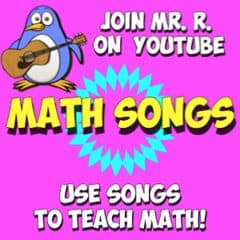# Mr. R.’s World of Math

## base ten detectives

Lesson: Base Ten Detectives
Lesson skills: number sense for place value, addition and subtraction skills

Materials:
base ten Blocks
hundreds chart
tens and ones paper (click button above to print .pdf)
base ten block sheets

Procedure:
1. Place some base-ten blocks on table and have students write the corresponding number on their tens and ones sheet.

For example: if the there are 2 tens rods and 3 unit cubes, they will write a 2 in the ten’s column and a 3 in the one’s column (They may also identify the number on the 100s chart to help them connect the number with standard written numbers)

2. Cover the base ten blocks with a sheet of paper, and show a new amount of base ten blocks.

For example: show 5 unit cubes

3. Tell students you will put the new set of blocks under the paper with the first set of blocks. Students will have to be detectives to come up with, and write the total number of cubes that are under the paper without seeing them. (they can use their tens and ones paper or hundreds charts to help)

4. After students are done figuring their answers, remove the paper and count blocks- focus on the number of tens rods and unit cubes.

Notes: start simple by adding an amount of unit cubes that do not go into the next ten’s place. Progress to adding greater numbers of unit cubes (such as 27 + 7) and tens rods as students get more adept.
Challenges:
Work across tens or hundreds.
Removal of base ten blocks from under the paper, instead of adding them is conceptually more challenging.